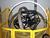## Need a little VB.Net help -2Tags:
Random
Random Number Generator
VB
VB.NET

We need a VB programmer to turn my rough shot at a function into VB.Net and put it through it's paces to see how it works, code is below in discussion.

.. earlier thread question Need a little VB.Net help. TheFinder was attempting to find a good solution to a lottery number picking problem. He needed to pick 6 numbers, each from 1 to 50 but no two duplicating. The six numbers had to total to a target value input by the user as the six digits of an earlier lottery ticket. I developed an algorithm (in GetSix below) that I believe will give a quick and simple solution to the problem. Unfortunately, I’m not VB.Net adept and I don’t have VB. If someone would like to fix up this code and give us a little idea of the performance (time and/or number of loops), that would be interesting.

Software/Hardware used:
VB.Net

Thanks. We'll let you know when a new response is added.

## Discuss This Question: 2 Replies

Thanks. We'll let you know when a new response is added.
•```Public Function GetSix(ByVal I1 As Integer, ByVal I2 As Integer, ByVal I3 As Integer, _
ByVal I4 As Integer, ByVal I5 As Integer, ByVal I6 As Integer, _
ByRef N1 As Integer, ByRef N2 As Integer, ByRef N3 As Integer, _
ByRef N4 As Integer, ByRef N5 As Integer, ByRef N6 As Integer, _
ByRef CountLoop As Integer) As Boolean

Dim Success As Boolean = True
Dim rnd1 As New Random()
Dim target As Integer
Dim maxTries As Integer= 25000
Dim maxTriesRule5 As Integer = 15000
Dim Rule1, Rule2, Rule3, Rule4, Rule5 as Boolean
Dim Rule4N1, Rule4N2, Rule4N3, Rule5N4, Rule5N5, RuleN6 = 0

CountLoop = 0
target = I1 + I2 + I3 + I4 + I5 + I6

If target < 21 ot target > 285 then
return false
endif

Do Until CountLoop > maxTries or Success

‘Loop
CountLoop += 1

N1 = rnd1.next(MAX( target - 240, 1), MIN( 50, target - 15) + 1 )
N2 = rnd1.next(MAX( target - N1 - 194, 1), MIN(50, target - 10 - N1) + 1)
N3 = rnd1.next(MAX( target - N1 - N2 - 147, 1), MIN(50, target - 6 - N1 - N2) + 1)
N4 = rnd1.next(MAX( target - N1 - N2 - N3 - 99, 1), MIN(50, target - 3 - N1 - N2 - N3) +1)
N5 = rnd1.next(MAX( target - N1 - N2 - N3 - N4 - 50, 1), MIN(50, target - 1 - N1 - N2 - N3 - N4) +1)
N6 = MAX( target - (N1 + N2 + N3 + N4 + N5), 1)

'----Rule 1, total of the inputs should equal the total of the outputs
Rule1 = (I1 + I2 + I3 + I4 + I5 + I6) = (N1 + N2 + N3 + N4 + N5 + N6)

'----Rule 2, N1, N2, N3, N4, N5, N6 must all be different
If Rule1 then
Rule2 =(N1 <> N2 And N1 <> N3 And N1 <> N4 And N1 <> N5 And _
N1 <> N6 And N2 <> N3 And N2 <> N4 And N2 <> N5 And _
N2 <> N6 And N3 <> N4 And N3 <> N5 And N3 <> N6 And _
N4 <> N5 And N4 <> N6 And N5 <> N6)
Endif

'----  Rule 3, N1 - N6 must be between 1 and 50
Rule3 = N6 > 0 and N6 <=50

'---- Rule 4, any digit cannot appear in the same location on the output as on the input N1 not equal to I1, N2 not equal to I2
if Rule1 and Rule2 and Rule3 then
Rule4 = (N1 <> I1 and N2 <> I2 and N3 <> I3 and N4 <> I4 and N5 <> I5 and N6 <> I6
Endif

'---- Rule 5, any digit cannot appear in the list if this is possible
if Rule1 and Rule3 and Rule3 and Rule4 and CountLoop < maxTriesRule5 then

Rule5 =(N1 <> I2 And N1 <> I3 And N1 <> I4 And N1 <> I5 And N1 <> I6 And_
N2 <> I1 And N2 <> I3 And N2 <> I4 And N2 <> I5 AND N2 <> I6 And_
N3 <> I1 And N3 <> I2 And N3 <> I4 And N3 <> I5 And N3 <> I6 And_
N4 <> I1 And N4 <> I2 And N4 <> I3 And N4 <> I5 And N4 <> I6 And_
N5 <> I1 And N5 <> I2 And N5 <> I3 And N5 <> I4 And N5 <> I6 And_
N6 <> I1 And N6 <> I2 And N6 <> I3 And N6 <> I4 And N6 <> I6 )
Endif

'---- test success
If   (Rule1 and Rule2 and Rule3 and Rule4 and Rule5) then
or (Rule1 and Rule2 and Rule3 and Rule4 and LoopCount> maxTriesRule5 )
Success = True
Else
Success = False then
If (Rule1 and Rule2 and Rule3 and Rule4) then  '--- save good rule 4 answer
Rule4N1 = N1
Rule4N2 = N2
Rule4N3 = N3
Rule4N4 = N4
Rule4N5 = N5
Rule4N6 = N6
Endif
Endif
If LoopCount > maxTriesRule5 and Rule4N1 <> 0 then '--- exhausted rule 5 use rule 4 answer
N1 = Rule4N1
N2 = Rule4N2
N3 = Rule4N3
N4 = Rule4N4
N5 = Rule4N5
N6 = Rule4N6
Success = True
Endif
Loop
loop
Return Success
End Function
```
report
•no edit on discussion here is a change Rule4N1 .. N6 are of type Integer .. Dim Rule4N1, Rule4N2, Rule4N3, Rule5N4, Rule5N5, RuleN6 As Integer = 0
report